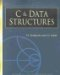# Memory Allocation

## DYNAMIC MEMORY ALLOCATIONS

### Introduction

You can use an array when you want to process data of the same data type and you know the size of the data. Sometimes you may want to process the data but you don't know what the size of the data is. An example of this is when you are reading from a file or keyboard and you want to process the values. In such a case, an array is not useful because you don't know what the dimension of the array should be. C has the facility of dynamic memory allocations. Using this, you can allocate the memory for your storage. The allocation is done at runtime. When your work is over, you can deallocate the memory. The allocation of memory is done using three functions: malloc, relloc, and calloc. The functions return the pointers to void, so it can be typecast to any data type, thus making the functions generic. These functions take the input as the size of memory requirement.

Program

#include #include main() { int *base; \ A int i; int cnt=0; int sum=0; printf("how many integers you have to store "); scanf("%d",&cnt); \ B base = (int *)malloc(cnt * sizeof(int)); \ C printf("the base of allocation is %16lu ",base); \ D if(!base) \ E printf("unable to allocate size "); else { for(int j=0;j

Explanation

1.
2. This program demonstrates the use of dynamic memory allocation for processing n integers where n is not defined at compilation time, but the user instead specifies the number of integers to be processed.
3.
4. The processing adds 5 to the value of each integer.
5.
6. Statement B reads how many integers you have to process.
7.
8. Statement C allocates memory for the required integers by using the function malloc.
9.
10. malloc takes the size in bytes as input.
11.
12. The size of the operator returns how many bytes can be occupied by one unit of the specified data type. The size of int returns two bytes. If you give the value cnt as 10 then it will allocate 20 bytes.
13.
14. malloc returns the pointer to void, which is typecast as a pointer to an integer. The value starts at the address of the memory from where allocations are done. The value is stored in the variable base, which is declared at statement A. If memory allocations cannot be done, the base will get the value 0, which can be tested using an if statement. The for loop F puts a value of 5 in the allocated memory. Note that the first value is stored in the location specified by the base and the next value is stored according to base +j. If the base is 100 and j is 1 then the value of base + 1 is 102, according to pointer arithmetic, and not 101, because this is a pointer to an integer and an integer occupies two bytes. You can retreive the value by using a pointer to an integer as specified by the for loop in statement G. After your work is over, you can return the memory using the function free. free takes a pointer to storage as input.
15.
16. You can again allocate more or less memory by using the function malloc. You can again allocate memory without deallocating previous memory as given by statement I. You can allocate the memory similarly to malloc by using the function calloc. calloc takes two arguments: total number of data and the size of each data.
17.

Points to Remember

You can allocate memory at runtime by using the function malloc. malloc allocates memory specified using an argument in terms of bytes, and returns the pointer to storage from where the memory is allocated. You can deallocate the memory by using the function free.

The prototypes of the function are available in the hidden files malloc.h.C & Data Structures (Charles River Media Computer Engineering)
ISBN: 1584503386
EAN: 2147483647
Year: 2006
Pages: 232